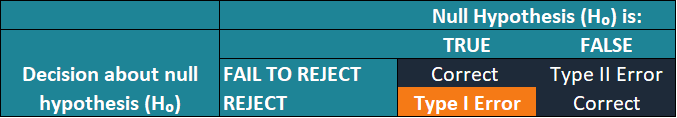# Type I Error

"False positive" error

## What is a Type I Error?

In statistical hypothesis testing, a Type I error is essentially the rejection of the true null hypothesis. The type I error is also known as the false positive error. In other words, it falsely infers the existence of a phenomenon that does not exist.

Note that the type I error does not imply that we erroneously accept the alternative hypothesis of an experiment.The probability of committing the type I error is measured by the significance level (α) of a hypothesis test. The significance level indicates the probability of erroneously rejecting the true null hypothesis. For instance, the significance level of 0.05 reveals that there is a 5% probability of rejecting the true null hypothesis.

### How to Avoid a Type I Error?

It is not possible to completely eliminate the probability of the type I error in hypothesis testing. However, there are opportunities to minimize the risks of obtaining results that contain a type I error.

One of the most common approaches to minimize the probability of getting a false positive error is to minimize the significance level of a hypothesis test. Since the significance level is chosen by a researcher, the level can be changed. For example, the significance level can be minimized to 1% (0.01). It will indicate that there is a 1% probability of incorrectly rejecting the null hypothesis.

However, lowering the significance level may lead to a situation wherein the results of the hypothesis test may not capture the true parameter or the true difference of the test.

### Example of a Type I Error

Sam is a financial analyst. He runs a hypothesis test to discover whether there is a difference in the average prices for large-cap and small-cap stocks.

In the test, Sam assumes that the null hypothesis is that the there is no difference in the average prices between the large-cap and small-cap stocks. Thus, his alternative hypothesis states that the difference between the average prices does exist.

For the significance level, Sam chooses 5%. It means that there is a 5% probability that his test will reject the null hypothesis when it is actually true.

If Sam’s test incurs a type I error, the results of the test will indicate that the difference in the average prices between the large-cap and small-cap stocks exists while there is no significant difference among the groups.